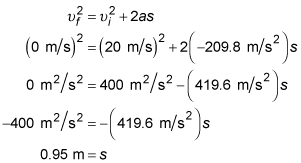##### Physics I: 501 Practice Problems For Dummies (+ Free Online Practice)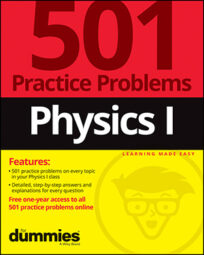In physics, you can calculate the trajectory of an object using the velocity-displacement formula. For example, given the mass and initial velocity of a Frisbee and the air resistance exerted on it, you can find the maximum height the Frisbee can reach.

Here are some practice questions that you can try.

## Practice questions

1. A baseball is thrown into the air, landing several meters away. What is the ball's vertical velocity at the highest point of its arc?

2. A 50-gram Frisbee is launched at an initial velocity of 20 meters per second straight up with its flat side parallel to the ground. The Frisbee experiences a constant force of air resistance of 10 newtons. What is the maximum height in meters that the Frisbee can reach above its original launch point?

The following are the answers to the practice questions:

1. 0 m/s

The highest point in a projectile's arc is the place where the projectile's vertical velocity changes from a positive (upward) value to a negative (downward) one. Therefore, its vertical velocity at the top must be 0 meters per second (the only number between positives and negatives). At the top of its arc, the baseball is no longer moving upward and is about to start moving downward; it is stationary with respect to the vertical axis of motion and has a velocity of 0 meters per second.

2. 0.95 m

First convert the mass into "correct" units: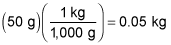After the Frisbee is launched, two forces act on it, both pointing down: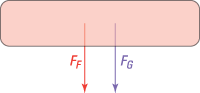Use the force form of Newton's second law to calculate the net acceleration on the Frisbee: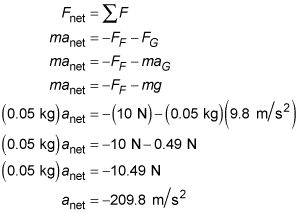Now use the velocity-displacement formula to solve for the Frisbee's maximum height — that is, the location where its vertical velocity equals 0 meters per second: